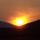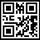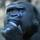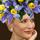## Social Question#### Care to test your mathematical intuition on this simple problem?

Asked by LostInParadise (29658) April 10th, 2020
11 responses
“Great Question” (3)

I just thought of this problem, and my initial guess was way off. See if you can come closer.

If you randomly choose two numbers from 1 to 100, what are the chances that they add up to a number from 1 to 100? Very likely? Very unlikely? What do you think?Follow this questionSend to a friend!Topics: , , ,
Observing members: 0Composing members: 0I am bad at math ,but will say very unlikely.

SQUEEKY2 (21203)“Great Answer” (1)if both numbers are chosen randomly, a 25 percent chance.

My reasoning:

Situation 1: If both numbers are > 50, then by definition the sum will be > 100

Situations 2 and 3: One number is > 50 and the other number <=50. so it’s a 50/50 chance of the sum > 100

Situation 4: Both numbers < 50 so the sum must be < 100.

I could be totally wrong.

elbanditoroso (31369)“Great Answer” (1)Intuition says 49% chance.

My reasoning: doesn’t the problem essentially distill into one random number between 2 and 200 being less than or equal to 100?

gorillapaws (28290)“Great Answer” (3)I like that reasoning ^^^, but doesn’t it fail to take into account the behavioral aspect? As worded, the question appears to imply that someone (you) is making the choice and not a random number generator.

The numbers aren’t actually chosen randomly if they’re chosen by a person. I’m wondering if a random person is more likely or less likely to pick relatively low numbers. Low, I’d think, meaning under 50.

If the numbers are machine-generated, then my intuitive guess is the same as @elbanditoroso.‘s

And here’s one of those weird little Fluther quirks. I wrote ’@’ followed by ‘elbanditoroso’ followed by an apostrophe-s (‘s) followed by a period. The software moved the period up to follow the name and tagged the ‘s on at the end.

Jeruba (52855)“Great Answer” (2)@Jeruba Good point. The wording is ambiguous. I assume the “you” is being used informally and would require the person to actually use a mechanism for deriving randomness (like 100-sided dice, or a pseduorandom number generator).

gorillapaws (28290)“Great Answer” (0)Sorry for any confusion. I thought that random would imply that they were not chosen deliberately

@gorillapaws got it right. Here is a similar way of thinking about it. The average value of the first number is (1 + 100)/2 = 101/2. Similarly for the second number, so their sum should average 101/2 + 101/2 = 101. A small portion of the numbers add up to 101. Of the remainder, as many add up to a sum less than 101 as add up to a sum over 101, so the probability of a sum from 1 to 100 is the first case, which would be just under a half.

Here is the math for getting an exact answer. There are 100×100 ways of choosing 2 numbers from 1 to 100.

Now let’s consider the cases where the sum is 1 to 100. If the first number is 1, the second can be any of the 99 numbers from 1 to 99. If the first number is 2, the second can be from 1 to 98. Continuing this way, the total number of cases is the sum of the numbers from 1 to 99. The formula for the sum of the numbers from 1 to n is n(n+1)/2, giving us 99×100/2 = 99×50.

The probability is therefore 99×50/100×100 = 99/100×50/100=0.99×0.50 = 0.495

LostInParadise (29658)“Great Answer” (0)Here is a solution that builds on the original intuition.

For each case of numbers a and b from 1 to 100 with sum s1 = a + b , choose the numbers (101 – a) and (101 – b) with sum s2 = 202 – (a+b). s1 + s2 = 202. If s1 < 101 then s2 > 101 and if s1 > 101 then s2 < 101. This pairing means that the number of sums < 101 equals the number of sums > 101, justifying our intuition.

The total number of sums is 100×100=10,000. Of these, each number x from 1 to 100 can be combined with one other number y such that x+y=101. Subtracting this from 10,000 gives 10,000 – 100 = 9900. Half of these add up to 1 to 100, giving 9900/2 = 4950. The probability is 4950/10,000 = 0.495.

LostInParadise (29658)“Great Answer” (0)@elbanditoroso , I have been thinking about your approach. Consider this extension of it.

Both numbers go from 1 to 100. Break this into two regions for each number, 1—> 50 and 51—>100. If both numbers are 1—>50 that is acceptable and if both are 51—>100 those are all unacceptable. So we have removed two equal size regions, one good and one bad.

We now have left one number 1—>50 and the other 51—>100. Break each of these regions in two. We have 1—>25 and 26—> 50 on the one hand and 51—>75 and 76—>100 on the other. 1—>25 combined with 51—>75 is good. 26—>50 and 75—>100 is an equal size bad region. We are left with two cases: one number being 1—>25 and the other 76—>100 and one number being 26—>50 and the other being 51—>75.

We could continue breaking regions in two and getting more and more smaller regions. We run into trouble with regions of odd numbered length, but this approach at least suggests that there are as many good cases as bad cases, so the probability would be about ½.

LostInParadise (29658)“Great Answer” (0)@LostInParadise As I was writing down my theory, I knew it was going to be a low estimate. My thinking wasn’t exactly what you wrote above, but it was along those same lines.

elbanditoroso (31369)“Great Answer” (1)I coded up a Monte Carlo simulation and ran 10million runs. 49.5%.

gorillapaws (28290)“Great Answer” (1)I just thought of this way of visualizing the problem.

On a coordinate plane, form a square through the points (1,1), (1,100), (100,1) and (100,100). The points (x,y) represent the two numbers selected.

The points where x+y = 101 lie on the diagonal of the square through (1,100) and (100,1). The points below the diagonal are where x+y < 101, that is x + y < =100. It is easy to see by symmetry that they are half the points excluding those on the diagonal.

The calculation follows easily. The total number of points in the square is 100×100=10,000, and there are 100 points on the diagonal, giving the number of points below the diagonal as (10,000 – 100)/2 , and we get 0.495 as before.

LostInParadise (29658)“Great Answer” (0)